International
Tables for
Crystallography
Volume F
Crystallography of biological macromolecules
Edited by M. G. Rossmann and E. Arnold

International Tables for Crystallography (2006). Vol. F, ch. 2.1, pp. 61-62   | 1 | 2 |

## Section 2.1.9. The Patterson function

J. Drentha*

aLaboratory of Biophysical Chemistry, University of Groningen, Nijenborgh 4, 9747 AG Groningen, The Netherlands
Correspondence e-mail: j.drenth@chem.rug.nl

### 2.1.9. The Patterson function

| top | pdf |

In 1934, A. L. Patterson presented a method for locating the atomic positions in not too complicated molecules without knowledge of the phase angles (Patterson, 1934). The method involves the calculation of the Patterson function,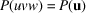: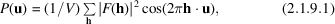or, written as an exponential function,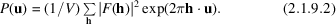Equations (2.1.9.1)and (2.1.9.2)give the same result, because in the definition of P(u) anomalous dispersion is neglected, resulting in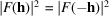. Comparison with equations (2.1.7.3)and (2.1.7.6)shows that the Patterson function P(u) is a Fourier summation with coefficientsinstead of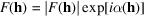. The periodicity, and thus the unit cell, are the same for the electron density and the Patterson function. For the Patterson function, many authors prefer to use u rather than r as the position vector.

The fundamental advantage of Patterson's discovery is that, in contrast to the calculation of, no phase information is needed for calculating P(u).

The Patterson map can be obtained directly after the intensities of the reflections have been measured and corrected. However, what kind of information does it provide? This can be understood from an alternative expression for the Patterson function: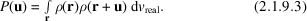Equation (2.1.9.3)leads to the same result as equation (2.1.9.1), as can be proved easily by substituting expression (2.1.7.3) for ρ in the right-hand side of equation (2.1.9.3).On the right-hand side of the equation, the electron densityat position r in the unit cell is multiplied by the electron density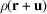at position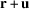; the integration is over all vectors r in the unit cell. The result of the integration is that the Patterson map will show peaks at the end of vectors u between atoms in the unit cell of the structure; all these Patterson vectors start at the origin of the Patterson cell. This can best be understood with a simple example. In Fig. 2.1.9.1,a two-dimensional unit cell is drawn containing only two atoms (1 and 2). To calculate the Patterson map, a vector u must be moved through this cell, and, according to equation (2.1.9.3),for every position and orientation of u, the electron densities at the beginning and at the end of u must be multiplied. It is clear that this product will generally be zero unless the length and the orientation of u are such that it begins in atom 1 and ends in atom 2, or the other way around. If so, there is a peak in the Patterson map at the end of vector u and at the end of vector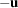, implying that the Patterson map is always centrosymmetric. The origin itself, where vector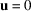, always has a high peak because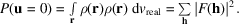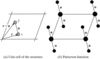Figure 2.1.9.1 | top | pdf |(a) A two-dimensional unit cell with two atoms. (b) The corresponding Patterson function. Reproduced with permission from Drenth (1999). Copyright (1999) Springer-Verlag.

The origin peak is equal to the sum of the squared local electron densities. The height of each non-origin peak is proportional to the product ofand. This is an important feature in the isomorphous replacement method for protein-structure determination, in which the heavy-atom positions are derived from a difference Patterson calculated with coefficients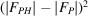, where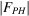is the structure-factor amplitude of the heavy-atom derivative and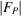is that of the native protein (see Part 12). The vectors between the heavy atoms are the most prominent features in such a map.

The number of peaks in a Patterson map increases much faster than the number of atoms. For n atoms in the real unit cell, there are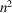Patterson peaks, n of them superimposed at the origin, and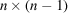elsewhere in the Patterson cell. Because the atomic electron densities cover a certain region and the width of a Patterson peak at u is roughly the sum of the widths of the atoms connected by u, overlap of peaks is a real problem in the interpretation of a Patterson map. It can almost only be done for unit cells with a restricted number of atoms unless some extra information is available. For crystals of macromolecules, it is certainly impossible to derive the structure from an interpretation of the Patterson map.

The situation can be improved through sharpening the Patterson peaks by simulating the atoms as point scatterers. This can be achieved by replacing the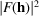values with modified intensities which, on average, do not decrease with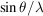. For instance, suitable intensities for this purpose are the squared normalized structure-factor amplitudes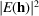(Section 2.1.4.6), the average of which is 1 at all. A disadvantage of sharpening to point peaks is the occurrence of diffraction ripples around the sharp peaks, induced by truncation of the Fourier series in equation (2.1.9.1).Therefore, modified intensities corresponding to less sharpened peaks are sometimes used [IT B (2001), Chapter 2.3, pp. 236–237]. Diffraction ripples that seriously disturb the Patterson map are generated by the high origin peak, and, particularly for sharpened maps, it is advisable to remove this peak. This implies that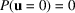[equation (2.1.9.1)].It is easy to verify that this requires coefficients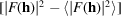for themap and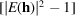for themap. Note that the term foris omitted and that the average ofmust be taken for the appropriateregion.

The symmetry in a Patterson map is related to the symmetry in the electron-density map, but it is not necessarily the same. For instance, screw axes in the real cell become non-screw axes in the Patterson cell, because all interatomic vectors start at the origin. It is possible, however, to distinguish between screw axes and non-screw axes by the concentration of peaks in the Patterson map. For instance, the consequence of a twofold symmetry axis along b is the presence of a large number of peaks in the (u0w) plane of the Patterson map. For a screw axis with translationalong b, the peaks lie in the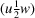plane. Such planes are called Harker planes (Harker, 1936). Peaks in Harker planes usually form the start of the interpretation of a Patterson map. Harker lines result from mirror planes, which do not occur in macromolecular crystal structures of biological origin.

Despite the improvements that can be made to the Patterson function, for structures containing atoms of nearly equal weight its complete interpretation can only be achieved for a restricted number of atoms per cell unless some extra information is available. Nowadays, most structure determinations of small compounds are based on direct methods for phase determination. However, these may fail for structures showing strong regularity. In these cases, Patterson interpretation is used as an alternative tool, sometimes in combination with direct methods. It is interesting to see that the value of the Patterson function has shifted from the small-compound field to macromolecular crystallography, where it plays an extremely useful role:

 (1) in the isomorphous replacement method, the positions of the very limited number of heavy atoms attached to the macromolecule can be derived from a difference Patterson map, as mentioned earlier in this section; (2) anomalous scatterers can be located by calculating a Patterson map with coefficients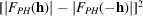, in which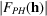is the structure-factor amplitude of the protein containing the anomalous scatterer; (3) molecular replacement is based on the property that the Patterson map is a map of vectors between atoms in the real structure, combined with the fact that such a vector map is (apart from a rotation) similar for two homologous structures: the unknown and a known model structure.

### References

International Tables for Crystallography (2001). Vol. B. Reciprocal space, edited by U. Shmueli. Dordrecht: Kluwer Academic Publishers.Google Scholar
Harker, D. (1936). The application of the three-dimensional Patterson method and the crystal structures of proustite, Ag3AsS3, and pyrargyrite, Ag3SbS3. J. Chem. Phys. 4, 381–390.Google Scholar
Patterson, A. L. (1934). A Fourier series method for the determination of the components of interatomic distances in crystals. Phys. Rev. 46, 372–376.Google Scholar